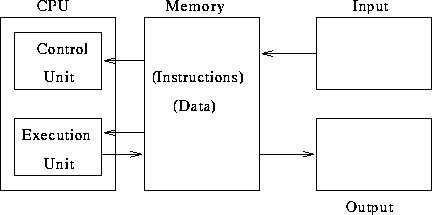# Computers, Program Execution, and Data Representation

Tom Kelliher, CS 102

Jan. 25, 1999

# Administrivia

### Announcements

First quiz on Friday.

Introductory exercises.

Survey

### Outline

1. Program execution.

2. A model computer.

3. Data representation.

### Coming Up

MS Word --- bring the workbook.

# Program Execution

Consider the following simple program:

``` 1:   let sum = 0
2:   print "How many numbers? "
3:   read count
4:   let loopCount = count
5:   if loopCount equals 0 goto 11
6:   print "Next number: "
7:   read input
8:   let sum = sum + input
9:   let loopCount = loopCount - 1
10:   goto 5
11:   let average = sum / count
12:   print "The average is:", average, "."
13:   end
```
1. Where do we begin?

2. After completing one step, where do we proceed?

3. Operations: assignment, arithmetic, decision, branch, I/O.

4. Operands: Variables, constants (numeric and string).

# The Basic Parts of a Computer

A block diagram:1. What is memory? How does it differ from storage?

2. What parts of a PC are considered input devices? Output devices? Both?

# Data Representation

1. Computers use the binary system. Why?

2. Binary digits.

3. Bits, bytes, words.

4. Memory locations: cells with addresses.

5. Converting between binary and decimal:
1. Binary to decimal: Consider some examples. 1, 10, 100, 110. What to do in general?

2. Decimal to binary: We need a little algorithm.
```read decimalNumber
while decimalNumber does not equal 0
{
let remainder be the remainder of decimalNumber / 2
let decimalNumber be the integer quotient of decimalNumber / 2
print remainder to the left of any other digits printed
}
```

6. How do we represent characters? ASCII code:
1. A: 01000001

2. 4: 00110100

7. How does the computer know if a memory location contains numbers, characters, variables, or instructions?

Thomas P. Kelliher
Sun Jan 24 18:45:59 EST 1999
Tom Kelliher